Orlík hydroelectric plant

The Orlík hydroelectric power plant, built in 1954-1961, consists of four Kaplan turbines. For each of them, the water with a flow rate of Q = 150 m3/s is supplied with a flow rate of h = 70.5 m at full power.
a) What is the total installed power of the power plant at efficiency n = 87%?
b) For the fast charging of electric vehicles overnight, power consumption up to P0 = 11kW is required. How many would electric cars cover power plant power?
c) How many days of full power operation corresponds to the delivered energy E = 398GWh

Result

P =  361.018 MW
n =  32820
d =  45.9

Solution:Leave us a comment of this math problem and its solution (i.e. if it is still somewhat unclear...):Be the first to comment!To solve this verbal math problem are needed these knowledge from mathematics:

Do you know the volume and unit volume, and want to convert volume units? Do you want to convert mass units?

Next similar math problems:

1. Cylindrical tank 2If a cylindrical tank with volume is used 12320cm raised to the power of 3 and base 28cm is used to store water. How many liters of water can it hold?
2. Friction coefficientWhat is the weight of a car when it moves on a horizontal road at a speed of v = 50 km/h at engine power P = 7 kW? The friction coefficient is 0.07
3. Solid in waterThe solid weighs in air 11.8 g and in water 10 g. Calculate the density of the solid.
4. Water flowHow much water flow in pipe with a diameter of 16 cm in 1 hour if the water velocity is 2.5 m/s?
5. Shot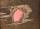Shot with a mass 43 g flying at 256 m/s penetrates into the wood to a depth 25 cm. What is the average force of resistance of wood?
6. G forcesCalculate deceleration of car (as multiple of gravitational acceleration g = 9.81 m/s2) which occurs when a car in a frontal collision slows down uniformly from a speed 111 km/h to 0 km/h in 1.2 meters trajectory.
7. Aircraft nose downHow long will fall airliner from a height of 10000 m at speed 1,000 km/h?
8. CarAt what horizontal distance reaches the car weight m = 753 kg speed v = 74 km/h when the car engine develops a tensile force F = 3061 N. (Neglect resistance of the environment.)
9. FlywheelFlywheel turns 450 rev/min (RPM). Determine the magnitude of the normal acceleration of the flywheel point which are at a distance of 10 cm from the rotation axis.
10. Gold horseshoe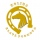Calculate the volume of a gold horseshoe that weighs 750g.
11. Iron cast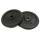What is the weight of cast iron with volume 3575 cubic centimeters. The density of the cast iron is 7600 kg/m3
12. Cuprum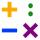From 1600 mm long sheet of copper plate 2 mm thickness we have separated over the whole length the belt weighing 6000 g. Calculate belt width if 1 dm3 copper weighs 8.9 kg.
13. Clock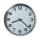How many hours are 15 days?
14. GravitationFrom the top of the 80m high tower, the body is thrown horizontally with an initial speed of 15 m/s. At what time and at what distance from the foot of the tower does the body hit the horizontal surface of the Earth? (use g = 10 ms-2)
15. Free fall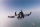How long does the stone fall freely into a depth of 80m? What speed will it hit the bottom of the abyss?
16. Free fallThe free fall body has gone 10m in the last 0.5s. Find the body speed at the moment of impact.
17. Bulb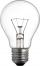A 60 W bulb consumes 0.12 kWh of electricity. How long did she stand light without a break?